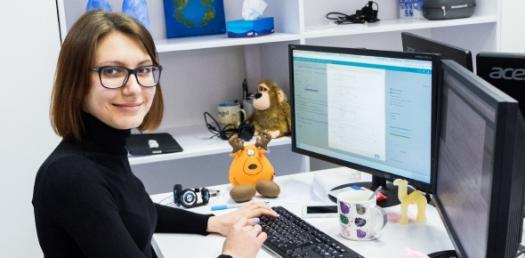# Computer Logic 2

10 Questions | Total Attempts: 251Settings.

Related Topics
• 1.
A logic gate that gives an output of TRUE only if both inputs are true
• A.

NOT gate

• B.

OR gate

• C.

AND gate

• 2.
An inverter gate
• A.

NOT gate

• B.

OR gate

• C.

AND gate

• 3.
What is the smallest binary number that can be represented in an 8-bit register?
• A.

0000 0000

• B.

1111 1111

• C.

255

• 4.
What is the largest unsigned binary number that can be represented in an 8-bit register?
• A.

0000 0000

• B.

1111 1111

• C.

255

• 5.
Give the two's complement of 010011001
• A.

10110111

• B.

10110110

• C.

1

• 6.
Add the following binar numbers: 1010 + 0010
• A.

1000

• B.

1110

• C.

1100

• 7.
Computer registers hold binary numbers.  Binary numbers can represent
• A.

Numbers and characters

• B.

Numbers only

• C.

Characters only

• 8.
What is an overflow error?
• A.

An error that occurs when a logic circuit can only output a 1

• B.

An error that occurs when a value is too large to fit in the available register

• C.

A programming error

• 9.
The one's complement of 01101110 is
• A.

11101110

• B.

01101111

• C.

10010001

• 10.
What is the smallest two's complement that can be stored in an 8-bit register? (Give the decimal equivalent of this number)
• A.

-128

• B.

0

• C.

-127